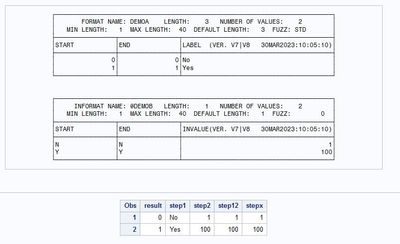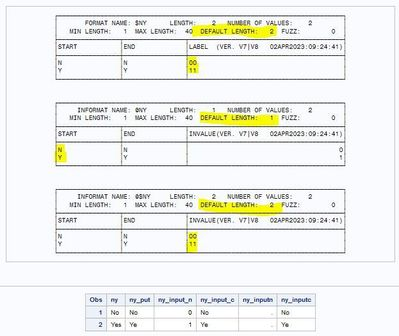## input function

Hi,

I'm really puzzled here.

I would expect step2/step12 would be set to missing, the solution being stepx but my output says differently 🤔

How could SAS is able to map No to 1 knowing that only N is in the informat.

``````
proc format fmtlib;
value demoa    0 ='No'
1 ='Yes';
invalue demob 'N'=1
'Y'=100;
select demoa @demob;
run;

data demo;
result=0; output;
result=1; output;
run;

data demo;
set demo;

step1 =put(result,demoa.);
step2 =input(step1,demob.);
step12=input(put(result,demoa.),demob.);

stepx =input(put(result,demoa1.),demob.);

put 'STEP 1' step1;
put 'STEP 2' step2;
run;

proc print data=demo;
run;
``````1 ACCEPTED SOLUTION

Accepted Solutions

## Re: input function

Since the possible arguments to your informat all have the length 1, the default length is also (implicitly) defined as 1, so it will always read only the first character of the string (unless you set another length when using the informat).

5 REPLIES 5

## Re: input function

Since the possible arguments to your informat all have the length 1, the default length is also (implicitly) defined as 1, so it will always read only the first character of the string (unless you set another length when using the informat).

## Re: input function

Thank you so much @Kurt_Bremser

I'm never thought about it as a possibility.

## Re: input function

Dear @Kurt_Bremser

I was rethinking at this input thing.

- For numeric and character formats, the default width is the format decode (label) width.

- For a numeric informat, the default width would then be the informat code (start/end).

1/ So I was wondering what is the default width for character informat. It seems to be the informat decode width.

2/ I was also wondering on whether inputn would work the same way as input+numeric informat. And it doesn't.

Actually inputn works the way I was expecting it to work. ny_inputn is set to missing

``````proc format fmtlib;
value   \$ny 'N'='00'
'Y'='11';
invalue ny  'N'=0
'Y'=1;
invalue \$ny 'N'='00'
'Y'='11';
run;

data demo;
length ny \$3;
ny='No'; output;
ny='Yes'; output;
run;

data demo;
set demo;
ny_put=put(ny,\$ny.);
ny_input_n=input(ny,ny.);
ny_input_c=input(ny,\$ny.);
ny_inputn=inputn(ny,'ny');
ny_inputc=inputc(ny,'\$ny');
run;

proc print data=demo;
run;``````## Re: input function

There is a big difference between the PUT and INPUT functions on the one side and the PUTN, PUTC, INPUTN and INPUTC functions on the other.

While all other functions expect numeric or character expressions as arguments, PUT and INPUT expect a format name, which is neither numeric nor character. While the N/C functions evaluate the second argument on their own during data step execution, for PUT and INPUT the data step compiler does this during compilation and then (IMO) implements the same mechanism used in PUT and INPUT statements. This (for me) explains the difference. PUT and INPUT are rather "compiler directives" than "normal functions".

While the INPUTN functions evaluates the whole first argument, the INPUT acts like the statement and reads the next X characters from the "input stream", where X is the width of the format.

An inconsistency, but explainable. Maybe an insider ( @ChrisHemedinger , can you call out to a developer?) can shed more light on this.

## Re: input function

You seem to have discovered that the INPUTN() function does not use the defualt width when the format specification does not provide one.

So just make sure to specify the width when you use inputn().

`` ny_inputn=inputn(ny,'ny1.');``

Or make sure you only give it one character if that is all you want it to read.

`` ny_inputn=inputn(char(ny,1),'ny.');``

Note that like the INPUT() function then INPUTN() function does not care if the informat width is larger than the length of the string being read. So this code works fine:

```61   data want;
62     string='123';
63     num1=input(string,32.);
64     num2=inputn(string,'32.');
65     put string= \$quote. num1= num2=;
66   run;

string="123" num1=123 num2=123
```
Discussion stats
• 5 replies
• 338 views
• 2 likes
• 3 in conversation